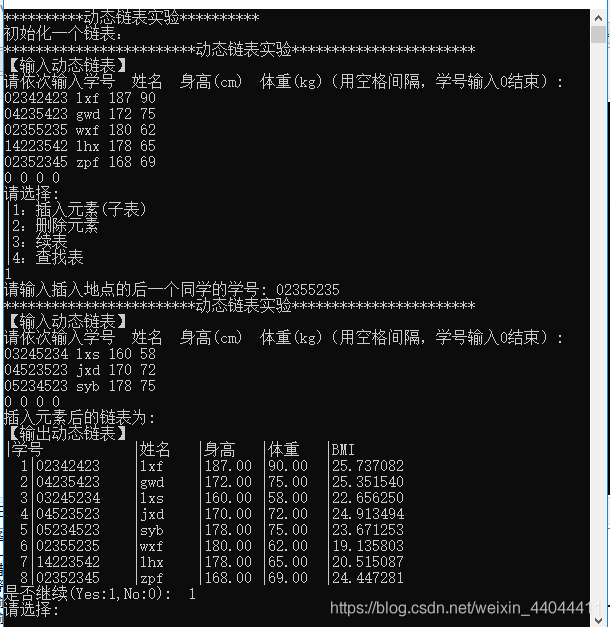﻿ C语言实现线性动态(单向)链表的示例代码_C 语言_脚本之家
C 语言# C语言实现线性动态(单向)链表的示例代码

## 什么是链表

```struct Student{
int ID;//学号
char;//姓名
int marks;//5门考试的成绩
struct Student *next;//指向下一个结构体的结构体指针
}
```

## 链表的操作

### 创建表

```struct Student * input()
{
printf("************************动态链表实验***********************\n【输入动态链表】\n");
printf("请依次输入学号	姓名  身高(cm)  体重(kg)（用空格间隔，学号输入0结束）:\n");
p2=p1=(struct Student *)malloc(LEN);//开辟内存
scanf("%d %s %f %f",&p1->ID,&p1->name,&p1->height,&p1->weight);
while(p1->ID!=0)
{
p2->next=p1;
p2=p1;
p2->BMI=(float)p2->weight/(p2->height/100)/(p2->height/100);//求BMI指数
p1=(struct Student *)malloc(LEN);//开辟内存
scanf("%d %s %f %f",&p1->ID,&p1->name,&p1->height,&p1->weight);
}
p2->next=NULL;
}
```

### 删除元素

```struct Student *delate(struct Student *head,int stdID)
{
struct Student *p1,*p2;
{
return p1;
}//如果删除的是第一个元素，比较特殊，需要修改头指针，其余不动
//剩余几种情况都是修改next结构体指针
{
if(p1->ID==stdID)
{
p2->next=p1->next;//假设找到了需要删除的学生的学号，则让它上一个学生的指针指向跳过他的下一个学生
free(p1);
}
}
return NULL;//返回NULL代表没找到
}
```

### 插入元素

```struct Student *insert(struct Student *head,int stdID,struct Student *insertstd)
{
struct Student *p1,*p2,*p;
for(p=insertstd;p->next!=NULL;p=p->next);//找到insert链表的最后一个元素
{
return insertstd;
}

{
if(p1->ID==stdID)
{
p2->next=insertstd;
p->next=p1;
}
}
return NULL;
}
```

## 代码及运行结果

```#include <stdio.h>
#include <malloc.h>
#include <stdbool.h>
#define LEN sizeof(struct Student)//定义结构体变量的大小为符号常量LEN
struct Student{
int ID;//学号
char name;//姓名
float height;//身高
float weight;//体重
float BMI;//BMI指数，录入时不需要计算
struct Student *next;//指向下一个结构体
};
struct Student *input();//输入函数
struct Student *delate(struct Student *head,int stdID);//删除一个元素，返回删除后表的头指针
struct Student *insert(struct Student *head,int stdID,struct Student *insertstd);//返回插入元素（子表）后的头指针
struct Student *isexist(struct Student *head,int stdID);
int main()
{
struct Student *present;//当前链表的头指针
int choice;
bool next;
int stdID;
/*
1：插入一个元素
2：删除一个元素
3：续表
4：查找表
*/
printf("**********动态链表实验**********\n初始化一个链表：\n");
present=input();//当前的链表指针
do{
printf("请选择:\n|1：插入元素(子表)\n|2：删除元素\n|3：续表\n|4：查找表\n");
scanf("%d",&choice);
switch(choice)
{
case 1:
printf("请输入插入地点的后一个同学的学号: ");
scanf("%d",&stdID);
if(isexist(present,stdID)==NULL)
{
printf("该学生不存在!\n");
break;//退出switch语句
}
present=insert(present,stdID,input());
printf("插入元素后的链表为:\n");
output(present);
break;
case 2:
printf("请输入删除元素的学号:  ");
scanf("%d",&stdID);
if(isexist(present,stdID)==NULL)
{
printf("该学生不存在!\n");
break;//退出switch语句
}
present=delate(present,stdID);
printf("删除后的链表为:\n");
output(present);
break;
case 3:
append(present);
printf("续表后的链表为：\n");
output(present);
break;
case 4:
printf("当前链表为：\n");
output(present);
break;
}
printf("是否继续(Yes:1,No:0):  ");
scanf("%d",&next);
fflush(stdin);
}while(next);

return 0;
}
struct Student * input()
{
printf("************************动态链表实验***********************\n【输入动态链表】\n");
printf("请依次输入学号	姓名  身高(cm)  体重(kg)（用空格间隔，学号输入0结束）:\n");
p2=p1=(struct Student *)malloc(LEN);//开辟内存
scanf("%d %s %f %f",&p1->ID,&p1->name,&p1->height,&p1->weight);
while(p1->ID!=0)
{
p2->next=p1;
p2=p1;
p2->BMI=(float)p2->weight/(p2->height/100)/(p2->height/100);//求BMI指数
p1=(struct Student *)malloc(LEN);//开辟内存
scanf("%d %s %f %f",&p1->ID,&p1->name,&p1->height,&p1->weight);
}
p2->next=NULL;
}
{
struct Student *p;
int num=1;
printf("【输出动态链表】\n");
printf("|学号\t\t|姓名\t|身高\t|体重\t|BMI\n");
while(p!=NULL)
{
printf("%3d|%08d\t|%s\t|%5.2f\t|%5.2f\t|%lf\n",num++,p->ID,p->name,p->height,p->weight,p->BMI);
p=p->next;
}
}
struct Student *delate(struct Student *head,int stdID)
{
struct Student *p1,*p2;
{
return p1;
}//如果删除的是第一个元素，比较特殊，需要修改头指针，其余不动
//剩余几种情况都是修改next结构体指针
{
if(p1->ID==stdID)
{
p2->next=p1->next;//假设找到了需要删除的学生的学号，则让它上一个学生的指针指向跳过他的下一个学生
free(p1);
}
}
return NULL;//返回NULL代表没找到
}
struct Student *insert(struct Student *head,int stdID,struct Student *insertstd)
{
struct Student *p1,*p2,*p;
for(p=insertstd;p->next!=NULL;p=p->next);//找到insert链表的最后一个元素
{
return insertstd;
}

{
if(p1->ID==stdID)
{
p2->next=insertstd;
p->next=p1;
}
}
return NULL;
}
{
struct Student *p;
p->next=input();//从input输入需要添加的元素，可以是1个或者多个
return 0;
}
struct Student *isexist(struct Student *head,int stdID)
{
struct Student *p;
{
if(p->ID==stdID)
{
return p;
}
}
return NULL;
}
```